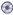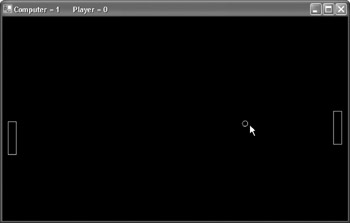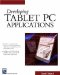# Pong GameDownload CD Content

## Overview

In this chapter, we continue in the general direction we started with in our last application by developing our first game. The game is a simple game of Pong (see Figure 26.1) using very basic drawing techniques and simple Artificial Intelligence (AI).Figure 26.1: The game we'll create is Pong.

 Note The source code for the projects are located on the CD-ROM in the PROJECTS folder. You can either type them in as you go or you can copy the projects from the CD-ROM to your hard drive for editing.

## Game Overview

All game projects, be it for the Tablet PC or a standard desktop PC, have a few concepts and are constructed in a similar manner. These are a few steps that are consistent among game projects:

1. Initialization: Initializes the key objects, such as starting locations of players, number of lives, score, direction, and so forth
2. Game Loop: Controls movement, score, and collisions for every frame of a game
3. Exit: Exits the game, stores high scores, asks if user wants to play again, and so on

Let's start a new Windows Forms application in VB and add the following variables to the project:

```Dim ball As Single
Dim x, y, dx, dy As Single
Dim blnGameOn As Boolean
Dim g As Graphics
Dim Computer As Integer
Dim Player As Integer
Dim MoveDown As Boolean
Dim MoveUp As Boolean```

## Game Loop

All of the action for our game takes place in a loop, but before we look through the procedure that will house the loop, let's take a minute to get the rest of the application out of the way.

The first step is to use the Form1_Activated event to start the threading for our game loop. Threads are a powerful abstraction for allowing parallelized operations. For example, graphical updates can happen while a different thread is performing computations. Conceptually, threads are pretty simple to understand, but they can get very complicated to manage from a programming standpoint. In this example, we'll have a very simple implementation:

```Private Sub Form1_Activated(ByVal sender As Object, ByVal e As System.EventArgs) Handles MyBase.Activated
t.Start()
End Sub```

The Form_Load event comes after the Form_Activated event. We use the Form_Load event to set the Text property to show the scores for the game (at this time 0 for the computer and the player), and we set the variables Player and Computer to 0.

Here is the procedure:

```Private Sub Form1_Load(ByVal sender As System.Object, ByVal e As System.EventArgs) Handles MyBase.Load
Me.Text = "Computer = " & "0" & " Player = " & "0"
Player = 0
Computer = 0
End Sub```

We will now implement a simple movement method similar to the virtual joystick we created in the previous chapter. We can test the pen's position and move the paddle depending on its position. Here is the code:

```Private Sub Form1_MouseMove(ByVal sender As Object, ByVal e As System.Windows.Forms.MouseEventArgs) Handles MyBase.MouseMove
If e.Y > (Me.Height / 2) + 50 Then
MoveDown = True
MoveUp = False
Exit Sub
ElseIf e.Y < (Me.Height / 2) - 50 Then
MoveUp = True
MoveDown = False
Exit Sub
Else
MoveUp = False```
``` End If
End Sub```

The final event we deal with before moving on to the loop thread is to set up what occurs when the form is closing. We set the Boolean variable blnGameOn equal to False so that the loop will end.

Here is the code:

```Private Sub Form1_Closing(ByVal sender As Object, ByVal e As System.ComponentModel.CancelEventArgs) Handles MyBase.Closing
blnGameOn = False
End Sub```

The remaining text in this chapter is used to go through the various parts of the game loop. It is the job of this loop to draw every frame of the game, creating the illusion of movement by repositioning each object after each frame is drawn.

The first part of the game procedure sets the size of the ball, the position of the ball, and the size and position of the computer controlled paddle (paddle1) and the player controlled paddle (paddle2).

Here is the code:

```Private Sub game()
Randomize()
ball = 10 'Size
x = Rnd() * (Me.Width - ball)
y = Rnd() * (Me.Height - 30 - ball)
dx = 1
dy = 1

g = Me.CreateGraphics

blnGameOn = True```

The game loop is a While loop, which continues executing as long as blnGameOn is equal to True. The loop consists of a series of steps, as follows:

1. Calculate the new position of objects.
2. Draw the object at the calculated location.
3. Provide the user with time to see the frame.
4. Delete the frame.

The objects in our game, a ball and two paddles, are moved according to four variables:

x: Controls horizontal position of the ball

y: Controls the vertical position of the ball

dx: Controls the direction and magnitude of movement of the object along the horizontal axis

dy: Controls the direction and magnitude of movement of the object along the vertical axis

To move the objects, we use the x, y, dx, and dy values of the objects with the following calculations:

x = x + dx

y = y + dy

After we calculate the new coordinate values, then we draw the object at this new location. There are a few additional things we need to check as we move the ball and paddles. That is, we need to calculate when there is a collision between the ball and either paddle, or the ball and the upper and lower bounds of the form, which results in reversing the direction of the ball. The last collision we need to watch is the collision between the ball and either end. This adds a point to the appropriate player and positions the items where they began. To reverse the direction of the object along its dx and dy, we just multiply these values by -1. Therefore if dy = 1 and the ball is moving downwards, then we can use dy = dy * -1 to change dy's value to -1, which in turn, makes the object move up.

There are many different algorithms involved in determining when two objects collide on a computer screen; we will look at one of the simpler, albeit inaccurate, ones. We calculate the distance between the centers of two objects on the screen. If it is less than the sum of the radius of the two objects, then the objects are going to collide.

If you remember back to the steps for our game loop, we have covered the first step. Now, it's time to draw the objects. For the ball, it's as simple as using the following:

`g.DrawEllipse(New Pen(Color.Orange), x, y, ball, ball`

Similarly, we can draw the paddles:

```g.DrawRectangle(New Pen(Color.White), xpaddle1, ypaddle1, paddleWidth, paddleHeight)

We now pause the application so the user can see how it appears on screen. We use the thread's Sleep method to delay the application. Next, we clear the graphics and then update the Text property of the form to reflect any new changes to the score.

Here is the complete procedure:

``` While (blnGameOn)
x = x + dx
y = y + dy
If ((x + ball) >= Me.Width) Then
dx = dx * -1
Computer = Computer + 1
x = Rnd() * (Me.Width - ball)
y = Rnd() * (Me.Height - 30 - ball)
dx = 1
dy = 1
End If

If (x <= 0) Then
dx = dx * -1
Player = Player + 1
x = Rnd() * (Me.Width - ball)
y = Rnd() * (Me.Height - 30 - ball)
dx = 1
dy = 1
End If

If ((y + ball) >= (Me.Height - 30) Or y <= 0) Then
dy = dy * -1
End If

g.DrawEllipse(New Pen(Color.Orange), x, y, ball, ball)

End If

End If

End If

If MoveDown Then
ElseIf MoveUp Then
Else
End If

If Math.Abs((x + ball / 2) - (xpaddle1 + paddleWidth / 2)) <
(ball / 2 + paddleWidth / 2) And Math.Abs((y + ball / 2) - (ypaddle1 +
paddleHeight / 2)) < (ball / 2 + paddleHeight / 2) Then
dx = dx * -1
ElseIf Math.Abs((x + ball / 2) - (xpaddle2 + paddleWidth / 2)) <
(ball / 2 + paddleWidth / 2) And Math.Abs((y + ball / 2) - (ypaddle2 +
paddleHeight / 2)) < (ball / 2 + paddleHeight / 2) Then
dx = dx * -1
End If

t.Sleep(10)

g.Clear(Color.Black)

Me.Text = "Computer = " & Computer.ToString & " Player = " & Player.ToString

End While

End Sub```

You can now test the application, which should look like Figure 26.2 when it is executed.Figure 26.2: The application is being executed.

## Summary

In this chapter, we built our first game for the Tablet PC. Although simple, it is a good introduction to some of the techniques you'll use with many of the games you can create. This game also used a technique similar to the virtual joystick for the movement of the player-controlled paddle. In Chapter 27, Not Quite a Magic Ball, we build another entertainment application that simulates a magic ball.Developing Tablet PC Applications (Charles River Media Programming)
ISBN: 1584502525
EAN: 2147483647
Year: 2003
Pages: 191